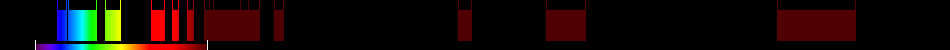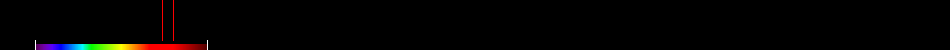# Information for Sensor and Index

## Formula

General Formula $\frac{\frac{{\mathrm{675nm}}}{{\mathrm{700nm}}}}{\frac{{\mathrm{r675}}}{{\mathrm{r700}}}}$ $\frac{\frac{{4}}{{5}}}{\frac{{\mathrm{r675}}}{{\mathrm{r700}}}}$ Automatic

## Sensor

Name Sentinel-2A 13 433-2280 10-60 98.6 ESA 2015-06-23 yes

## Index

Name Ratio Analysis of Reflectance Spectra A1 RARSa1 $\frac{\frac{{\mathrm{675nm}}}{{\mathrm{700nm}}}}{\frac{{\mathrm{r675}}}{{\mathrm{r700}}}}$ r=percentage reflectance at the specified wavelength in the reference releectance spectrum (in the present study this was the mean of 10 spectra of mature (i.e., presenescent) leaves with high pigment concentration) 675,700 Original formula

## References

No results for the selected combination.

## Visualisation

SensorIndex